Next: Construction of probabilistic models Up: Ensemble learning Previous: Connection to coding

## EM and MAP

Expectation maximisation (EM) algorithm can be seen as a special case of ensemble learning. The set-up in EM is the following: Suppose we have a probability model. We observe x but y remains hidden. We would like to estimate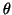with maximum likelihood, i.e., maximise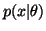w.r.t., but suppose the structure of the model is such that integration overis difficult, i.e., it is difficult to evaluate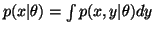.

What we do is take the cost function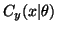and minimise it alternately with respect toand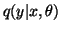. The ordinary EM algorithm will result whenhas a free form in which casewill be updated to be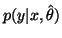, where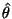is the current estimate of. The method is useful if integration over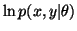is easy, which is often the case. This interpretation of EM was given by .

EM algorithm can suffer from overfitting because only point estimates for the parametersare used. Even worse is to use maximum a posterior (MAP) estimator where one finds theand y which maximise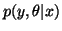. Unlike maximum likelihood estimation, MAP estimation is not invariant under reparametrisations of the model. This is because MAP estimation is sensitive to probability density which changes nonuniformly if the parameter space is changed nonlinearly.MAP estimation can be interpreted in ensemble learning framework as minimising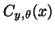and using delta-distribution as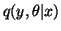. This makes the integral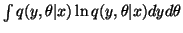infinite. It can be neglected when estimatingand y because it is constant with respect to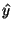and, but the infinity of the cost function shows that delta distribution, i.e. a point estimator, is a bad approximation for a posterior density.Next: Construction of probabilistic models Up: Ensemble learning Previous: Connection to coding
Harri Lappalainen
2000-03-03